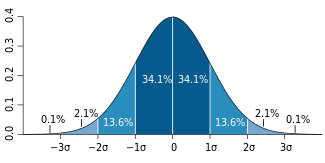## Friday, 23 June 2017

### Removing Outliers using stddev()

A colleague asked me about removing outliers from reports and I knew just the function to give a go.

Some time ago I had to prove that some data we had conformed to a normal distribution. Remember those from high school?A normal distrubition (bell curve)
That request was about 2007 and I ended up using stddev() and lpad() to produce a vertical shaped bell curve in SQL*Plus. That was pretty cool.

As for removing outliers, we can use this tool to exclude a small percentage of whatever data you have, so that it doesn't skew averages.

If you want to look at the code in context, I've created a test case on livesql.oracle.com

But as an inline reference, first I created a simple table, and populated it with a small set of random data between known range.
(I would like to add this is exactly what Tim Hall recently described here in regard to learning and asking questions.)

Importantly, I also inserted an outlier that was an order of magnitude higher than the rest.
My aim was to ignore this outlier in my SQL that calculated average.
```create table outlier_demo  (a number, val number);

insert into outlier_demo
select rownum -- we don't care
, round(dbms_random.value(20,50),1) -- some random numbers between 20 and 50
from dual
-- let's create 49 rows
connect by level < 50

insert into outlier_demo values (0,500)

select * from outlier_demo;

A       VAL
---     ----
1       27.9
2       42.5
3       49.7
4       22.3
5       42.3
6       20.2
7       33.9
8       46.8
...
48      45.7
49      21.8
0       500```
The average is higher than expected because of that outlier. I've included a column that calculates the average without the outlier, albiet hardcoded. Notice how close it is to the median? When considering usage statistics from APEX, I've often paid more attention to the median (most rows) than the average (affected by outliers).
```select count(*) c
-- what's our average?
,round(avg(val)) the_avg
-- what would the average be without the known outlier?
,round(avg(case when val < 500 then val end)) no_outlier
,round(stddev(val)) std
-- what's the median
,round(median(val)) the_median
from outlier_demo

C    THE_AVG NO_OUTLIER    STD   THE_MEDIAN
---  ------- ----------  -----   ----------
50        46         37     66           36
```
We can take the same function and get the result for every row, that's one way we can use it to filter outlier data.
```select a, val
-- what is 2 standard deviations away
-- provide result for each row
,round(2*stddev(val) over (order by null)) local_std
from outlier_demo```
Re-written as a from clause, we can now filter any rows that have a value higher than x standard deviations from the norm.
```select count(*) c
,round(avg(val)) the_avg
,round(avg(case when val < 500 then val end)) no_outlier
,round(stddev(val)) new_stddev
,round(avg(local_std)) filter
from
(select a, val
-- what is 2 standard deviations away
-- provide result for each row
,round(2*stddev(val) over (order by null)) local_std
from outlier_demo
)
-- only average those values within two standard deviations
where val < local_std;

C   THE_AVG NO_OUTLIER  NEW_STDDEV  FILTER
--  ------- ----------  ----------  ------
49       37        37           9      132
```
This could be re-written in a form that may be more recognisable to some.
```select count(*) c
,avg(val) the_avg
,avg(case when val < 500 then val end) no_outlier
,stddev(val) std
from outlier_demo
where val < (select 2*stddev(val) from outlier_demo);
```
There are probably a number of variations on how to apply this solution, and possibly a few alternative methods.
It will probably depend on the SQL in your particular report.

This is not to say you should be ignoring outliers completely, but stddev() can help identify them where necessary. And it scales.

Thank Kate for this post.

ps - If you want to dive deeper, Jonathan Gennick (my patient book editor) has two in depth posts on the topic, with some diagrams to help understand the mathematical concepts.

Related post:
Filtering outliers from APEX workspace activity logs